Question

# When a 2 kg mass is hung on a vertical spring, it extends the spring by...

When a 2 kg mass is hung on a vertical spring, it extends the spring by 2.00 m.
A) What is the magnitude of the force on the mass due to the spring?
N

 Tries 0/2

B) What is the spring constant of the spring? (Assume g = 10m/s2).
N/m

 Tries 0/2

C) What is the angular velocity ω of the mass when it oscillates back and forth on this spring?
s-1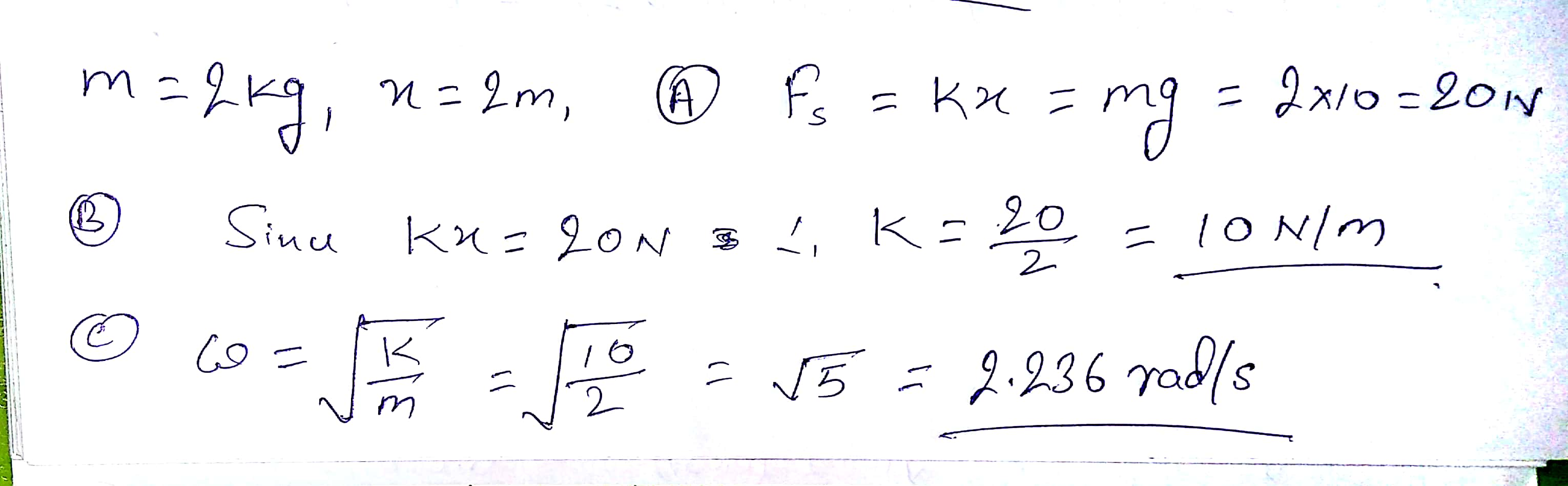#### Earn Coins

Coins can be redeemed for fabulous gifts.

Similar Homework Help Questions
• ### A block with mass m =6.6 kg is hung from a vertical spring. When the mass...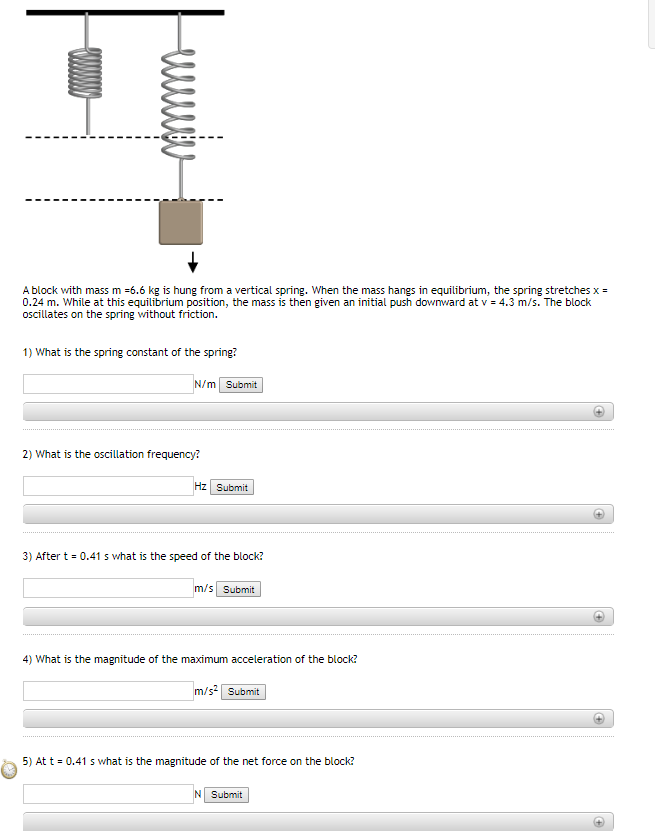A block with mass m =6.6 kg is hung from a vertical spring. When the mass hangs in equilibrium, the spring stretches x = 0.24 m. While at this equilibrium position, the mass is then given an initial push downward at v = 4.3 m/s. The block oscillates on the spring without friction. 1) What is the spring constant of the spring? N/m Submit 2) What is the oscillation frequency? Hz Submit 3) After t = 0.41 s what is...

• ### A block with mass m =7.5 kg is hung from a vertical spring. When the mass...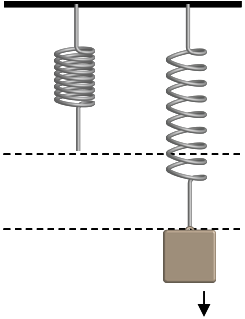A block with mass m =7.5 kg is hung from a vertical spring. When the mass hangs in equilibrium, the spring stretches x = 0.27 m. While at this equilibrium position, the mass is then given an initial push downward at v = 4.2 m/s. The block oscillates on the spring without friction. 3) After t = 0.32 s what is the speed of the block? 5) At t = 0.32 s what is the magnitude of the net force...

• ### A block with mass m =7.2 kg is hung from a vertical spring. When the mass...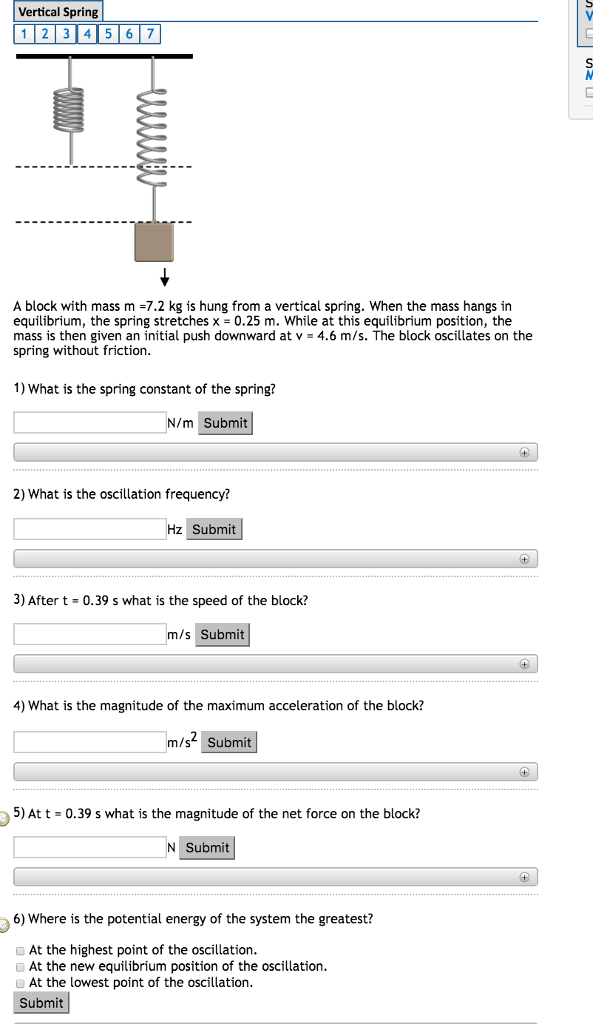A block with mass m =7.2 kg is hung from a vertical spring. When the mass hangs in equilibrium, the spring stretches x = 0.25 m. While at this equilibrium position, the mass is then given an initial push downward at v = 4.6 m/s. The block oscillates on the spring without friction. What is the spring constant of the spring? What is the oscillation frequency? After t = 0.39 s what is the speed of the block? What is...

• ### A block with mass m =6.2 kg is hung from a vertical spring. When the mass...A block with mass m =6.2 kg is hung from a vertical spring. When the mass hangs in equilibrium, the spring stretches x = 0.22 m. While at this equilibrium position, the mass is then given an initial push downward at v = 4.7 m/s. The block oscillates on the spring without friction. 1) What is the spring constant of the spring? 2) What is the oscillation frequency? 3) After t = 0.33 s what is the speed of the...

• ### Horkovember 21 Vertical Spring 34,056.7 A block with mass m =6.9 kg is hung from a...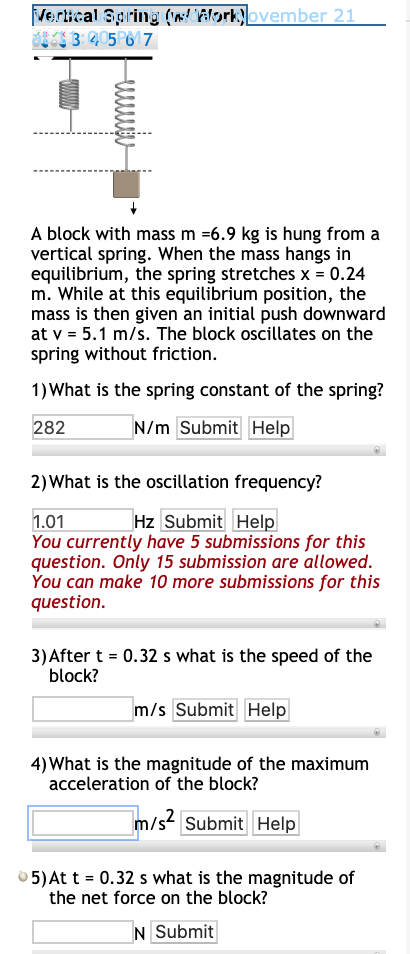Horkovember 21 Vertical Spring 34,056.7 A block with mass m =6.9 kg is hung from a vertical spring. When the mass hangs in equilibrium, the spring stretches x = 0.24 m. While at this equilibrium position, the mass is then given an initial push downward at v = 5.1 m/s. The block oscillates on the spring without friction. 1) What is the spring constant of the spring? 282 N/m Submit Help 2) What is the oscillation frequency? 1.01 Hz Submit...

• ### A block with mass m =7.3 kg is hung from a vertical spring. When the mass...A block with mass m =7.3 kg is hung from a vertical spring. When the mass hangs in equilibrium, the spring stretches x = 0.29 m. While at this equilibrium position, the mass is then given an initial push downward at v = 4.9 m/s. The block oscillates on the spring without friction. 1) What is the spring constant of the spring? 2) What is the oscillation frequency? 3) After t = 0.45 s what is the speed of the...

• ### A block with mass m =7.5 kg is hung from a vertical spring. When the mass...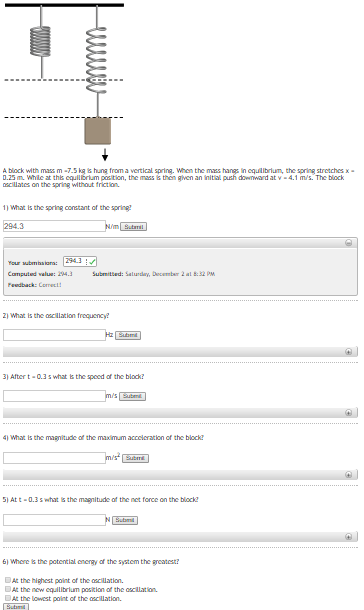A block with mass m =7.5 kg is hung from a vertical spring. When the mass hangs in equilibrium, the spring stretches x = 0.25 m. While at this equilibrium position, the mass is then given an initial push downward at v = 4.1 m/s. The block oscillates on the spring without friction. A block with mass m-7.5 ka hung from a vertical spring. Whon the ms hang in equilibrlum, the spring stretches x 0.25m. while at thk equErium pacition,...

• ### A block with mass m =7.5 kg is hung from a vertical spring. When the mass...

A block with mass m =7.5 kg is hung from a vertical spring. When the mass hangs in equilibrium, the spring stretches x = 0.25 m. While at this equilibrium position, the mass is then given an initial push downward at v = 4 m/s. The block oscillates on the spring without friction. 1) What is the spring constant of the spring? 294 N/m 2) What is the spring constant of the spring? 0.996 Hz 3) After t = 0.3...

• ### A block with mass m =7.1 kg is hung from a vertical spring. When the mass...A block with mass m =7.1 kg is hung from a vertical spring. When the mass hangs in equilibrium, the spring stretches x = 0.23 m. While at this equilibrium position, the mass is then given an initial push downward at v = 4.4 m/s. The block oscillates on the spring without friction. 1) What is the spring constant of the spring? N/m You currently have 4 submissions for this question. Only 10 submission are allowed. You can make 6...

• ### A block of mass 0.427 kg is hung from a vertical spring and allowed to reach...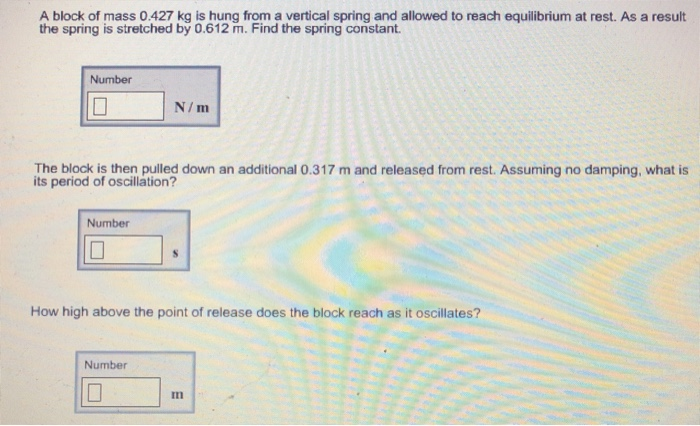A block of mass 0.427 kg is hung from a vertical spring and allowed to reach equilibrium at rest. As a result the spring is stretched by 0.612 m. Find the spring constant Number N/m The block is then pulled down an additional 0.317 m and released from rest. Assuming no damping, what is its period of oscillation? Number How high above the point of release does the block reach as it oscillates? Number In